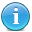# How Many Birds And Other Wildlife Are Killed By Cats In Your Community Calculator

We would prefer to call this calculator the “Why We Love Indoor Cats Calculator” because we love cats, but we also love wildlife. This calculator estimates the number of wildlife kills that occur both weekly and annually based on estimated cat populations using either the most current (hourly) US population estimate from the US Census or the number you enter. It then calculates the current number of households that are cat owners, the percentage of cats that are allowed outdoors, and the number of feral cats. The default numbers change hourly because they are based on US human population estimates. If you want to use this calculator to get estimates for your region, find your population stats at the US Census http://quickfacts.census.gov/qfd/index.html.

channel->item as \$post) { //echo "
" . \$post->title; //\$title = \$post->title; //get part of string //\$a = \$title; //\$c = explode(" ", \$a); //\$b = \$c; if (empty(\$_POST['number']) || \$_POST['number'] == 0) { //\$d = \$c; \$pop = 328653775; } else { \$pop = \$_POST['number']; } //echo "
" . \$_POST['number']; //if(\$b == "US") { //\$pop = str_replace("Estimate:", "", \$d); //\$pop = str_replace(",", "", \$pop); \$house = \$pop/2.64; \$s1 = 'As of ' . date("m-d-Y") . ' it is estimated that there are currently ' . number_format(\$pop) . ' people in your community, equaling ' . number_format(round(\$house)) . ' households.'; \$chouse = \$house * .304; \$s4 = '' . number_format(round(\$chouse)) . ' of households in your community own a cat.'; \$c1house = \$chouse * 2.1; \$c2house = \$c1house * .36; \$s6 = '

#### Number Of Owned Cats

There is an average of 2.1 cats per cat-owning household, totalling an estimated ' . number_format(round(\$c1house)) . ' cats currently in your community. ' . number_format(round(\$c2house)) . ' (36%) of owned cats are are allowed access to the outdoors.'; \$feral = \$house * .05; \$feral2 = \$c1house; \$s7 = '

#### Number Of Feral Cats

There are an estimated ' . number_format(round(\$feral)) . ' to ' . number_format(round(\$feral2)) . ' feral cats in your community'; \$cats = \$c2house + \$feral; //\$cats = \$feral; \$s8 = '

#### Number Of Outdoor Cats

This totals ' . number_format(round(\$cats)) . ' outdoor cats in your community. (*using the low end of the feral cat population estimate)'; \$kcats = \$cats *.333333; \$week = \$kcats * 2; \$year = \$week * 52; \$s9 = '

#### Number Of Wildlife Kills

Recent research shows that one in 3 cats kills wildlife, at a rate of 2 kills per week. This means that ' . number_format(round(\$kcats)) . ' cats kill ' . number_format(round(\$week)) . ' wildlife per week, or ' . number_format(round(\$year)) . ' wildlife per year.'; \$s10 = 'Of these kills, 27% are birds, 65% are mammals, and 8% are reptiles and amphibians. This means that
• ' . number_format(round(\$year*.27)) . ' birds are killed each year.
• ' . number_format(round(\$year*.65)) . ' mammals are killed each year.
• ' . number_format(round(\$year*.1)) . ' reptiles and amphibians are killed each year.'; \$s11 = 'A cat\'s instinct to hunt is separate from its hunger drive. Seventy (70%), ' . number_format(round(\$year*.7)) . ', of wildlife killed by cats are not eaten.'; \$s12 = 'The economic impact just from bird kills alone is estimated to be \$' . number_format(round(\$year*.27*30)) . '.'; //} //} ?>
# of People• Category: Pets